# JP3654470B2 - Echo canceling method for subband multi-channel audio communication conference - Google Patents

## Info

Publication number
JP3654470B2
JP3654470B2 JP24352496A JP24352496A JP3654470B2 JP 3654470 B2 JP3654470 B2 JP 3654470B2 JP 24352496 A JP24352496 A JP 24352496A JP 24352496 A JP24352496 A JP 24352496A JP 3654470 B2 JP3654470 B2 JP 3654470B2
Authority
JP
Japan
Prior art keywords
echo
signal
channel
subband
signals
Prior art date
Legal status (The legal status is an assumption and is not a legal conclusion. Google has not performed a legal analysis and makes no representation as to the accuracy of the status listed.)
Application number
JP24352496A
Other languages
Japanese (ja)
Other versions
JPH1093680A (en
Inventor

Original Assignee

Priority date (The priority date is an assumption and is not a legal conclusion. Google has not performed a legal analysis and makes no representation as to the accuracy of the date listed.)
Filing date
Publication date
Application filed by 日本電信電話株式会社 filed Critical 日本電信電話株式会社
Priority to JP24352496A priority Critical patent/JP3654470B2/en
Publication of JPH1093680A publication Critical patent/JPH1093680A/en
Application granted granted Critical
Publication of JP3654470B2 publication Critical patent/JP3654470B2/en
Anticipated expiration legal-status Critical
Expired - Lifetime legal-status Critical Current

• 230000000875 corresponding Effects 0.000 claims abstract description 27
• 230000002087 whitening Effects 0.000 claims description 15
• 230000005540 biological transmission Effects 0.000 claims description 6
• 230000002194 synthesizing Effects 0.000 claims description 4
• 230000015556 catabolic process Effects 0.000 claims description 2
• 230000004059 degradation Effects 0.000 claims description 2
• 238000006731 degradation reactions Methods 0.000 claims description 2
• 238000001514 detection method Methods 0.000 claims 2
• 230000001629 suppression Effects 0.000 claims 2
• 238000000034 methods Methods 0.000 description 22
• 238000004364 calculation methods Methods 0.000 description 15
• 230000000694 effects Effects 0.000 description 14
• 238000004458 analytical methods Methods 0.000 description 7
• 238000005094 computer simulation Methods 0.000 description 2
• 238000004519 manufacturing process Methods 0.000 description 1
• 238000003786 synthesis reactions Methods 0.000 description 1

## Images

•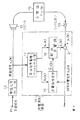••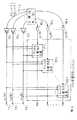•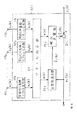•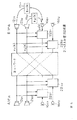••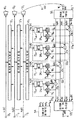•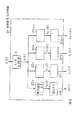•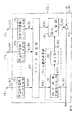•••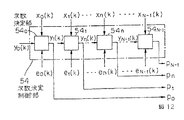•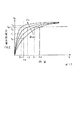•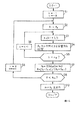•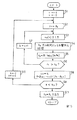•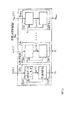•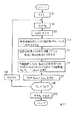•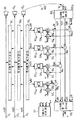•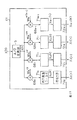•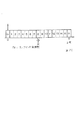•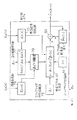•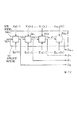•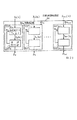•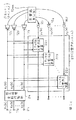•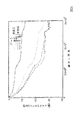••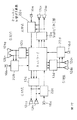## Classifications

• HELECTRICITY
• H04ELECTRIC COMMUNICATION TECHNIQUE
• H04MTELEPHONIC COMMUNICATION
• H04M9/00Interconnection arrangements not involving centralised switching
• H04M9/08Two-way loud-speaking telephone systems with means for suppressing echoes or otherwise conditioning for one or other direction of traffic
• H04M9/082Two-way loud-speaking telephone systems with means for suppressing echoes or otherwise conditioning for one or other direction of traffic using echo cancellers
• HELECTRICITY
• H04ELECTRIC COMMUNICATION TECHNIQUE
• H04MTELEPHONIC COMMUNICATION
• H04M1/00Substation equipment, e.g. for use by subscribers; Analogous equipment at exchanges
• H04M1/60Substation equipment, e.g. for use by subscribers; Analogous equipment at exchanges including speech amplifiers

## Abstract

In a subband echo cancellation for a multichannel teleconference, received signals x1(k), x2(k), . . . , xI(k) of each channel are divided into N subband signals, an echo y(k) picked up by a microphone 16j after propagation over an echo path is divided into N subband signals y0(k), . . . ,yN-1(k), and vectors each composed of a time sequence of subband received signals x1(k), . . . , xI(k) are combined for each corresponding subband. The combined vector and an echo cancellation error signal in the corresponding subband are input into an estimation part 19n, wherein a cross-correlation variation component is extracted. The extracted component is used as an adjustment vector to iteratively adjust the impulse response of an estimated echo path. The combined vector is applied to an estimated echo path 18n formed by the adjusted value to obtain an echo replica. An echo cancellation error signal en(k) is calculated from the echo replica and a subband echo yn(k).

## Description


[Industrial application fields]
The present invention relates to an echo cancellation method for multi-channel audio communication conferences that eliminates room echo signals that cause acoustic feedback and cause hearing problems in a communication conference system having a multi-channel playback system, and in particular for each divided frequency band. The present invention relates to an echo cancellation method for a subband multi-channel audio communication conference in which an estimated impulse response of a pseudo echo path is corrected by a projection algorithm or the like.

[Prior art]
Echo cancellation for 1 channel
There is an echo canceling device in order to provide a loudspeaking call system with excellent simultaneous call performance and low echo.
First, an echo canceling method and apparatus configuration of an echo canceling apparatus for one channel will be described with reference to FIG. In a voice call, the received signal obtained by the other party's utterance at the receiving terminal 11 is reproduced from the speaker (reproducer) 12 as it is, and before being sent to the speaker 12, the amplitude of the received signal, the magnitude of the power, etc. In some cases, the reception signal processing unit 13 that automatically adjusts the gain in response to the signal is inserted, and the reception signal is subjected to some processing and then reproduced from the speaker 12. Therefore, in this specification, the received signal x1(k) is not limited to the received signal itself from the other party, and refers to the processed received signal when the received signal processing unit 13 is inserted. k represents discrete time. The echo canceler 14 receives the received signal x1The echo signal y obtained by (k) being collected by the microphone (sound collector) 16 through the echo path 15 from the speaker 12.1Delete (k). Where the echo signal y1(k) represents the impulse response of the echo path 15 at time k as h.11(k, l)
It can be modeled as that obtained by a convolution operation such as L is the number of taps, and is a constant set in advance corresponding to the reverberation time of the echo path 15. First, in the received signal accumulation / vector generation unit 17, the received signal x1Accumulate (k) up to L-1 time past. The accumulated signal is the received signal vectorx 1(k), ie
x 1(k) = [x1(k), x1(k-1), ..., x1(k−L + 1)]T                (2)
Is output as However, *TRepresents the transpose of a vector. In the pseudo echo path 18, the received signal vector of equation (2)x 1(k) and the pseudo echo obtained from the echo path estimation unit 19
Road vectorh^11Inner product operation with (k)
y ^1(k) =h^11 T(k)x 1(k) (3)
As a result, the pseudo echo signal y ^1Generate (k). This inner product operation is equivalent to a convolution operation as shown in Equation (1). The echo path estimation unit 19 uses a pseudo echo path vector used in the pseudo echo path 18.h^11Generate (k).

Here, the impulse response h of the echo path 15 from the speaker 12 to the microphone 1611Since (k, l) fluctuates due to changes in the sound field due to movement of people or objects, for example, the pseudo-echo path vector follows this temporal variation.h^11It is necessary to change (k). This
In the configuration example, the echo canceling device 14 is configured using an adaptive FIR filter. The most common algorithm used for echo path estimation is the NLMS algorithm (learning identification method). In the NLMS algorithm, the received signal vector at time kx 1(k) and residual echo signal e1(k), that is, the subtractor 21 and the microphone 16
Output y1From (k) pseudo echo signal y ^1minus (k)
e1(k) = y1(k) −y ^1(k) (4)
From the pseudo echo path vector used at time k + 1h^11(k + 1)
However, μ is called a step size parameter and is used for adjustment of the adaptive operation in the range of 0 <μ <2. By repeating the above processing, the echo path estimation unit 19 gradually becomes a pseudo echo path vector.h^11(k) is the impulse response h of the true echo path 151
1An echo path vector with each element of (k, l) time seriesh 11(k), ie
h 11(k) = [h11(k, 0), h11(k, 1), ..., h11(k, L-1)]T            (6)
And as a result, the residual echo signal e in equation (4)1(k) can be reduced.

Currently, the most effective algorithm used for the echo path estimation is a projection algorithm or an ES projection algorithm. The projection algorithm is based on the idea of improving the convergence speed for a correlated signal such as speech by removing the autocorrelation of the input signal inside the algorithm. Removing the autocorrelation component means that the signal is whitened in the time domain. Details of the projection algorithm can be found in the document “Ozeki, Umeda,“ Adaptive Filter Algorithm Using Orthogonal Projection to Affine Subspace and Its Properties ”, IEICE Journal (A), J67-A, pp. 126-132. , (Sho 59-2) ".

In general, the p-th projection algorithm uses the past p input signal vectors.x(k
),x(k-1),…,xCorrect output y (k), y (k-1), ..., y (k-
p + 1) to obtain a pseudo echo path vectorhCorrect ^ (k). That is,
x T(k)h^ (K + 1) = y (k)
x T(k-1)h^ (K + 1) = y (k-1) (7)

x T(k-p + 1)h^ (K + 1) = y (k-p + 1)
Satisfyh^ (K + 1) is calculated. However,
x(k) = [x (k), x (k-1), ..., x (k−L + 1)]T                  (8)
It is. When the number of equations p is less than the number of unknowns (the number of taps) L, the solution of the simultaneous equations of equation (7)h^ (K + 1) is indefinite. So, the size of the correctionh^ (K + 1) −
hModify so that ^ (k) ‖ is minimized. The p-th projection algorithm is
However,
+  : Generalized inverse matrix
-1  : Inverse matrix
It is expressed.β(k) is the p-ary simultaneous linear equation
[X T(k)X(k))β(k) =e(k) (15)
Is the solution. Using a small positive constant δ to avoid instability in inverse matrix operations
[X T(k)X(k) + δI]β(k) =e(k) (15) '
It is good. However,IIs the identity matrix. The second term on the right side of equation (9) is the modified vector
The pseudo echo path vector is sequentially updated with the correction vector. In equation (9)X(k)β(k) represents the process of removing the autocorrelation of the input signal.
This means signal whitening processing in the time domain. That is, it can be said that the projection algorithm increases the correction speed of the impulse response by whitening the input signal in the time domain. Several high-speed arithmetic methods that reduce the amount of calculation have been proposed for the projection algorithm, and details thereof are described in JP-A-7-31535 and JP-A-7-92980. The case where the negative time input / output is zero and p is infinite corresponds to the RLS algorithm.

The ES projection algorithm is a combination of the projection algorithm and an ES algorithm that focuses on the fluctuation characteristics of the echo path, and can provide an echo canceller that has a higher convergence speed than the projection algorithm. The p-th ES projection algorithm is
However,
A= Diag [α1, α2,…, ΑL]: Step size matrix
αi= Α0λi-1(i = 1,2,…, L)
λ: Attenuation rate of impulse response fluctuation (0 <λ <1)
μ: Second step size (scalar amount)
It is expressed.β(k) is the p-ary simultaneous linear equation
[X T(k)AX(k))β(k) =e(k) (17)
Is the solution. Using a small positive constant δ to avoid instability in inverse matrix operations
[X T(k)AX(k) + δI]β(k) =e(k) (17) '
It is good. However,IIs the identity matrix.

If the pseudo echo path 18 is composed of a digital FIR filter, its filter coefficienth^11(k) is the impulse response of the indoor echo path 15h 11Simulate (k) directly
It has become. Therefore, the magnitude of the filter coefficient correction required according to the fluctuation of the echo path 15 is the echo path impulse response.h 11It agrees with the fluctuation amount of (k). there,
Step size matrix representing correction width in filter coefficient correction operationAIs Impa
Weighted with the time-varying characteristics of the Lus response. In general, an impulse response fluctuation amount in a room sound field is expressed as an exponential function using an attenuation factor λ. Step size matrixADiagonal component ofl(l = 1, 2,..., L) is α as l increases as shown in FIG. 2A.0
To exponentially decay with the same slope as the exponential decay characteristic of the impulse response and asymptotically approach zero. This algorithm uses the acoustic knowledge that when the impulse response of the room reverberation path fluctuates due to movement of people or objects, the fluctuation amount (difference of the impulse response) exponentially attenuates at the same attenuation rate as the impulse response. Is. It is possible to provide an echo canceller having a high convergence speed by correcting the initial coefficient of the impulse response having a large variation with a large step and the later coefficient of the impulse response having a small variation with a small step.

When the echo canceling device is configured by a plurality of DSP (Digital Signal Processor) chips, as shown in FIG.lThe exponential decay curve is approximated stepwise, and a constant α for each chip.lSet. Thereby, the ES projection algorithm can be realized with a calculation amount and a storage capacity substantially equal to those of the conventional projection algorithm. Details of the ES projection algorithm are disclosed in Japanese Patent Laid-Open No. 5-244043, S. Makino and Y. Kaneda, “Exponentially weighted step-size projection algorithm for acoustic echo cancellers”, Trans. IEICE Japan, vol. E75-A, no. 11, pp.1500-1508, Nov.1992.

From the above,hThe NLMS algorithm used in the conventional device with ^ (k) update formula
Compared with the case of the LSMS and the case of the (ES) projection algorithm, the expression (5) by the NLMS algorithmh^ (K) is the input signal vectorxin the direction of (k)
Updated. On the other hand, in the formulas (9) and (16) by the (ES) projection algorithm,h^ (K
) Is the second term on the right side of equation (9) and (16).
v(k) = β1 x(k) + β2 x(k-1) + ... + βp x(k-p + 1) (18)
Anyway, thisvdirection of (k), ie, input signal vectorx(k) to past
Input signal vectorx(k-1),…,xVector with all correlations with (k-p + 1) removed
Updated in the direction of That is, the correction vectorv(k) is the past signal in the input signal
So that the signal is removed as much as possible.1~ ΒpDecide.

Further, conventionally, a signal is divided into a plurality of frequency bands, and for each frequency band, the filter coefficient of the pseudo echo path 18 based on the fluctuation of the echo path 15 is sequentially corrected by the NLMS algorithm or the like. A subband method is known in which the residuals are combined and output. For example, US Pat. No. 5,272,695, S. Gay and R. Mammone, “Fast converging subband acoustic echo cancellation using RAP on the WER DSP16A ”, Proc.ICASSP90, pp.1141-1144, Apr.1990, etc. By this subband method, the signal is flattened in the frequency domain, so-called whitening, and when the echo path changes The convergence speed in the estimation of the pseudo echo path filter coefficient is increased.

Echo cancellation for teleconferencing system
Now, in general I (>2) Channel playback system and J (>1) Elimination of reverberation in the case of a communication conference system constituted by a channel sound collection system is performed by a configuration as shown in FIG. That is, an I-channel echo canceller 22 that processes an I-input 1-output time-series signal between all reproduction-side I channels and one sound-collecting channel.1, 222, ..., 22JAre realized as an echo canceling system 23 connected to each other. In this case, I × J echo paths 15 in the entire systemij(1<i<I, 1<j<J) exists. An I channel echo canceling device 22 connected between all I channels on the reproduction side and one channel on the sound collection side, which are constituent units of this system.1, 222, ..., 22J4 is configured as shown in FIG. 4 by expanding the configuration of the echo canceller 14 shown in FIG. This is described in detail in, for example, the IEICE Transactions '86 / 10 Vol. J69-A No. 10 “Multichannel Adaptive Digital Filter”. Here, the sound collection side is the jth sound collection channel (1<j<J) I-channel echo canceller 22 connected tojthink of. J-th channel sound collector 16jThe reverberation signals collected at the replay channel 15 are the reception signals of the respective reproduction channels.1j~ 15IjTherefore, the reverberation path estimation is uniformly performed for any reproduction channel by using the same residual echo signal e.jA device for evaluating only (k) is required. First, for the reception signal of each reproduction channel, the reception signal accumulation / vector generation unit (171, 172, ..., 17I)
x 1(k) = [x1(k), x1(k-1), ..., x1(k−L1+1)]T               (19)
x 2(k) = [x2(k), x2(k-1), ..., x2(k−L2+1)]T               (20)
:
x I(k) = [xI(k), xI(k-1),…, xI(k−LI+1)]T              (twenty one)
Is generated. However, L1, L2,…, LIIs the number of taps and each echo path 151j, 152j,…, 15IjIt is a constant set in advance corresponding to the reverberation time. These vectors are converted by the vector combiner 24,
x(k) = [x 1 T(k),x 2 T(k), ...,x I T(k)]T               (twenty two)
Combine with. The echo path estimation unit 19j, Each pseudo echo path vector for simulating I echo paths between each reproduction channel and the j-th sound collection channelh^
1j(k),h^2j(k), ...,h^IjCombine (k)
Treat as. Pseudo echo path coupling vectorh^j(k) is updated by the NLMS algorithm
When using
It is done like this. Pseudo echo path 18jNow, the inner product operation
y ^j(k) =h^j T(k)x(k) (25)
Thus, the echo signal y collected in the jth sound collection channeljPseudo echo signal y ^ for (k)jGenerate (k). In this way, by combining vectors for each channel and treating them as one vector, the basic processing flow is the same as that of the one-channel echo canceller shown in FIG.

[Problems to be solved by the invention]
Of the shortcomings of the conventional echo canceling system used in the communication conference system composed of the I-channel reproduction system and the J-channel sound collection system, what the present invention intends to solve will be described along with a specific example. .
As shown in FIG. 5, when the conventional echo canceling system is applied to a stereo audio conference apparatus that collects and plays back two channels between the points A and B, the echo path on the point B side changes completely. Even if not, there is a problem that the reverberation sound from the B point for the utterance increases every time the speaker moves or changes on the A point side. This is a problem that occurs because the echo path estimation is not performed correctly in the echo cancellation system on the B point side.

Therefore, in order to explain this problem, a device 22b connected to the first sound collection channel, which is one of the two-channel echo cancellation devices that are constituent units of the echo cancellation system at point B.1Pay attention to the behavior of. In the following, in order to clarify the operation of each reproduction channel, the elements for each reproduction channel of the combined vector used in the section of the prior art will be described in a specified form. A 2-channel received signal vector on the B point side expressed by a vector for each reproduction channel without using a combined vectorx 1(k) and
x 2(k). True reverberation path 15 for each playback channel11, 15twenty oneThe echo road
Koutlh 11(k),h twenty oneIf (k), these echo paths 1511, 15twenty oneThrough
The echo signal y1(k)
y1(k) =h 11 T(k)x 1(k) +h twenty one T(k)x 2(k) (26)
It is expressed. On the other hand, the pseudo echo signal y ^ generated in the echo canceller1(k) is the simulated echo path generated in the deviceh^11(k) andh^twenty oneUsing (k),
y ^1(k) =h^11 T(k)x 1(k) +h^twenty one T(k)x 2(k) (27)
And obtained. When one speaker speaks from point A, this received signal vectorx 1(k) andx 2There is a very strong cross-correlation with (k).x 1(k) andx 2(
If there is a constant strong cross-correlation with k),
y ^1(k) = y1(k) (28)
As a solution of [h^11 T(k),h^twenty one T(k)] is innumerable,x
1(k) andx 2Subspace inherent to cross-correlation with (k)H xForm. For this reason, N
When using a general sequential error minimization algorithm such as the LMS algorithm,h^11 T(k),h^twenty one T(k)] is subspace from initial valueH xThe distance to
It converges to a small point and is generally true [h 11 T(k),h twenty one T(k)] does not converge.

For ease of explanation, a constant scalar value p1, p2And the original signal vectors(k)
The received signal vectorx 1(k),x 2(k) is
x 1(k) = p1 s(k),x 2(k) = p2 s(k) (29)
Is considered. [h^11 T(k),h^twenty one T(k)] can exist
H xIs
p1 h^11(k) + p2 h^twenty one(k) = p1 h 11(k) + p2 h twenty one(k) (30)
6A satisfying the above, and when adaptation is started from the initial value 0, the convergence point [h^11p T(k),h^21p T(k)]
Is obtained as follows. For this reason, the scalar value p1And p2When the ratio between and fluctuates, equation (30) is no longer satisfied, so the echo cannot be eliminated and the echo increases.

As can be seen from the above example, when a conventional echo canceling apparatus is applied to a communication conference system generally composed of an I-channel playback system and a J-channel sound collection system, the received signal between each channel is not received. When there is a cross-correlation, the echo path is not correctly estimated, which causes a problem that the echo increases every time the cross-correlation between received signals varies.

In order to solve this problem, it is possible to improve the convergence speed to the true value by extracting and using the information of the fluctuation of the cross-correlation between the reception signals of each channel using a projection algorithm or the like. Proposed in However, this proposed method, in which the impulse response in the entire frequency band is corrected by a projection algorithm, has a problem that the convergence speed is still slow. Further, in order to improve the convergence speed, a very high projection order is required, and there is a problem that the amount of calculation increases.

As described above, the projection algorithm and the ES projection algorithm increase the convergence speed by removing the autocorrelation of the input signal. On the other hand, the subband method of processing a signal by dividing it into a plurality of frequency bands increases the convergence speed by flattening (whitening) the input signal in the frequency domain. Here, it is not known at all how the subband configuration affects the cross-correlation. Rather, even if the subband method is applied to a stereo echo canceller (stereo echo canceller), the above-described problem of the stereo echo canceller has not been solved at all and has been considered useless. For this reason, the full-band method has been used for a long time.
An object of the present invention is to provide an echo cancellation method for multi-channel audio communication conference that solves the above-described problems in the conventional echo cancellation system for multi-channel audio communication conference.

[Means for Solving the Problems]
Prior to explaining the method of the present invention, the operation of the echo path estimation unit of the multi-channel echo canceller when the cross-correlation between multi-channel received signals fluctuates is based on a part of the concept of the present invention. To do.
For simplicity, the 2-channel stereo reception signal expressed by Equation (29)x 1(k) andx 2(
p) in Equation (29) as a case where the cross-correlation with k) fluctuates1, p2Are different from each other in q1, q2Consider an example that has changed. First, [h^11 T(k),h^twenty one T(k))
In the equations (31) and (32)h^11p T(k),h^21p Tconverge to (k)]. Then p1
And p2And q1And q2When changed toh^11p T(k),h^21p T(k)]
As the “expected value”, the shortest distance from it [h^11q T(k),h^21q T(k)]
Bundle. This operation is interpreted geometrically as shown in FIG. 6B. Where the convergence point (h^11q,h^21q) Is the convergence point (h^11p,h^21p) To straight lineh^11q
1+h^twenty oneq2=h 11q1+h twenty oneq2It becomes the intersection of the vertical line. Therefore figure
Filter coefficient error vector e in 6Bp, eqThe norm is generally ‖ep‖> ‖EqIt is self-evident that there is a relationship of jealousy. This generally means that the norm of the filter coefficient error vector becomes smaller each time the cross-correlation between received signals changes. In other words, fluctuations in cross-correlation between multi-channel received signals cause an increase in echoes when the echo path estimation is incorrect in the echo canceller, but after an infinite number of fluctuations, It can be understood that this is effective information that enables estimation of a true echo path in the path estimation unit.

The multi-channel echo cancellation method according to the present invention includes a first method, a second method, and a third method.
As a first method, a subband method is used in the multi-channel echo cancellation method. In the subband method, since the time interval between samples is widened by thinning, the information on the fluctuation of the cross correlation is emphasized. Of course, as in the case where the subband method is used for the one-channel echo cancellation method, the input signal is flattened (whitened) in the frequency domain at the same time. This improves the convergence speed of true value estimation.

As a second method, a correction vector is obtained from the residual echo signal and the received signal of each channel in the first method, and the impulse response estimation of each echo path is sequentially corrected using this correction vector. In the invention, the fluctuation component of the cross-correlation between the current received signals with respect to the cross-correlation of the past received signals between the channels is extracted, and this fluctuation component is used as the correction vector. As an example, an (ES) projection algorithm is used as a method of enhancing the cross-correlation variation between channels. In this way, when the (ES) projection algorithm is used for the subband multi-channel echo canceller, the number of taps is reduced by decimation in the subband method. The effects of emphasizing the fluctuations in the cross-correlation are synergized to produce an unexpected effect, and a very fast convergence speed can be obtained with a small projection order. Furthermore, the maximum effect can be obtained with a small amount of calculation by optimally setting the projection order in each frequency band.

As a third method, a function of actively changing the cross-correlation between received signals in the first method within a range that does not cause a sense of incongruity is added, and an acoustic signal is reproduced by each regenerator. The received signal to which the cross-correlation variation is added is used to derive a correction vector of the pseudo echo path vector. In addition, by optimally setting the added function to be different for each frequency band, it is possible to minimize deterioration in hearing.

DETAILED DESCRIPTION OF THE INVENTION
FIG. 7 shows an example of the functional configuration of an echo canceling apparatus to which the echo canceling method of the present invention using the first method and the second method is applied, and parts corresponding to those in FIG. Receive signal xi(k ′) is the speaker 12iAnd the frequency band dividing unit 51iN real signals x in each frequency bandin(k) is divided into (n = 0, 1,..., N−1). On the other hand, the speaker 12iPlayed sound from the echo path 15ijThrough the microphone 16jDue to the echo signal yjPicked up as (k '). This echo signal yj(k ′) is the frequency band dividing unit 52.jWithin the frequency band dividing unit 51iN real signals y by frequency band with the same division characteristics asjnDivided into (k). Thereafter, since j is the same process, j is omitted for simplicity.

Frequency band dividing unit 51iIn each frequency band divided by the received signal accumulation / vector generation unit 17in, Vector coupling unit 24n, Pseudo echo path 18n, Echo path estimation unit 19nI channel echo canceller 22 comprisingnAre provided for each of the simulated echo paths 18.nIs a pseudo echo signal y ^n(k) is generated, and the subtractor 21nThese pseudo echo signals y ^n(k) echo signal y in the corresponding frequency bandnEcho signal y by subtracting from (k)n(k) is deleted. Here, the pseudo echo path 18nNeeds to follow the time course of the echo path 15 and the residual en(k) = yn(k) −y ^nAn estimation unit 19 using a projection algorithm or an ES projection algorithm so that (k) approaches 0nPseudo echo path vector byh^n(k) is estimated sequentially, and the pseudo-echo path 18nWill be corrected
Therefore, the optimal echo cancellation is always maintained. Error signal (residual signal) for each frequency band en(k) is a frequency band synthesizer 53.jThen, the error signal e (k ′) in all frequency bands is synthesized and output to the transmission end 4.

FIG. 8 shows an internal functional configuration of the frequency band dividing unit 51, and the received signal x (t) is sampled by the A / D converter 5, and the bandpass filter 20.nDue to the bandwidth limitation. Alternatively, the band pass filter 20nInstead of using the SSB (Single Side Band) method described in the document Crochiere and Rabiner, “Multirate Digital Signal Processing”, Englewood Cliffs, NJ: Prentice-Hall, pp. 52-56, 1983, Band-limited real signal xn(k ′) can be obtained. Band-limited real signal xn(k ′) is thinned out at a thinning rate R, and a real signal xn(k). Hereafter, this signal x of each divided frequency bandn(k) is called a subband received signal. The frequency band dividing unit 52 for the echo signal y (k ′) is also configured in the same manner as the frequency band dividing unit 51 of FIG.n(k) is called a subband echo signal.

In the subband method, the frequency bandwidth is narrowed, and the time interval between samples is widened by thinning, so that the information on fluctuations in cross-correlation is emphasized. For this reason, the convergence speed to the true value is increased.
Further, in the second method, as described above, information on fluctuations in cross-correlation between received signals of each channel is extracted and used. FIG. 9 shows an echo canceling device 22 in each frequency band.jIndicates. Parts corresponding to those in FIG. In the present invention, each echo canceller 221~ 22jIs provided with a cross-correlation fluctuation component extraction unit 31. In the cross-correlation fluctuation component extraction unit 31, the received signal combination vectorx(k) and residual echo signal ejInformation of (k)
And the autocorrelation removal vector, that is, the correction vectorvGenerate (k). This
Vector ofv(k)x(k) is a combination of multi-channel signal vectors with high cross-correlation
In the case of a vector, a component in which cross-correlation between channels does not change is removed, and a vector in which a fluctuation component of cross-correlation is emphasized is obtained. In the correction unit 32, the current estimated echo path vectorh^jFor (k), the correction vectorv(k)
Combined vectorh^j(k) is updated, and this is replaced with the pseudo echo path 18.jOutput to.

In the configuration of the conventional apparatus, received signal vectors between channels are combined and handled. For this reason, the cross-correlation between the reception signals of each channel appears in the autocorrelation that is the correlation between the reception signal combination vector and the past reception signal combination vector. Therefore, in order to remove the components whose cross-correlation is not changed and to extract the components whose cross-correlation is changed from the received signal of each channel, the auto-correlation of the received signal combination vector is reduced, that is, the received signal is combined. A technique for removing vector autocorrelation may be used. As such a method, a method using a projection algorithm, an RLS algorithm, or linear prediction in a one-channel echo canceller has been proposed. Among these methods, the linear prediction method performs linear prediction of a speech signal and removes autocorrelation of the speech signal using the linear prediction coefficient. The RLS algorithm can be regarded as a special case of the projection algorithm. Therefore, in the present invention, as an example of the most realistic method, a method for removing the autocorrelation of the received signal combined vector when the projection algorithm is used will be described.

FIG. 10 shows an estimation unit 19 for the nth frequency band.nP as an example of the internal functional configurationnNext ES projection algorithm (A=IWhen using the projection algorithm)
Show.
The received signal storage unit 31 stores the subband received signal xnEach time (k) is given, L is consecutive.nP consisting of individual subband received signalsnSubband signal vectors
And subband received signal matrix from these vectors
X n(k) = [x n(k),x n(k-1), ...,x n(k-pn+1)]
Is generated. LnIs the pseudo echo path 18 in the nth frequency band.nIs the number of taps of the FIR filter that constitutes. In these formulasx n(k),x n(k-1), ... are equations
This is a vector-combined vector given by (22). That is, the reception signal storage unit 31 stores the 1 to I channel subband reception signals.x n(k) is a vector combination
X n(k) is input.

However, in the nth frequency band, pnThe following projection algorithm or ES projection algorithm shall be executed. The step size matrix storage unit 32 stores the first step size matrix.A n= Diag [αn1, αn2,…, ΑnLn] Is memorized. Step size
matrixA nIs weighted by the fluctuation characteristics of the impulse response in the corresponding frequency band
It has been.

In the case of a general room, the amount of impulse response fluctuation in the nth frequency band is the attenuation rate γ.nIt is expressed as an exponential function using. In the autocorrelation calculating unit 33, the first step size matrixA nWeighted subband received signal matrix
X n(k) autocorrelation matrixX n(k)T A n X n(k) is calculated. This computed
Autocorrelation matrix and residual e from residual storage 34n(k) isβ n(k) Provided to the calculation unit 35
P of the following formulanFormer simultaneous linear equations
[X n(k)T A n X n(k)]β n(k) =e n(k) (33)
By solving for the constantβ nFind (k). Avoid instabilities in inverse matrix operations
In order to use a small positive constant δn instead of equation (33)
[X n(k)T A n X n(k) + δn I]β n(k) =e n(k) (33) '
May be used. However,IIs the identity matrix. Step size storage 36
Their second step size μnWhen,A n,X n(k),β n(k) is the correction vector
The correction vector supplied to the calculation unit 37
μn A n X n(k)β n(k) (34)
Is calculated, and the correction vector is supplied to the adder 38 and the L from the tap coefficient storage unit 39 is obtained.nPseudo-echo vector consisting of elementsh^nCorrected by adding to (k)
Simulated echo path vectorh^n(k + 1) is obtained. Calculation resulth^n(k + 1) is pseudo
Echo 18nAt the same time, the value of the tap coefficient storage unit 39 is updated.

Repeat the above operation for subband reception signal xnBy repeating each time (k) is given, the pseudo echo path 18nIs corrected sequentially according to the following equation (35), and the pseudo echo vectorh^n(k) is the impulse response of the true pseudo-echo path 15 in the nth frequency band.
h nApproaching (k).
What should be noted here is that the fluctuation of the cross-correlation varies depending on each frequency band, and is generally small in the low range and large in the high range. Considering this point, the projection order is increased in the frequency band where the cross-correlation fluctuation is small to increase the convergence speed, and the projection order is reduced in the frequency band where the cross-correlation fluctuation is large, thereby reducing the amount of computation. The convergence speed as a whole can be made uniform.

Another thing to note is the number of taps L in each band.nIs that the number is reduced by thinning. In the (ES) projection algorithm, the relationship between the order p and the convergence characteristic is affected by the tap number L. That is, when the tap number L is large, it is necessary to increase the order p in order to completely saturate the convergence speed, and when the tap number L is small, the convergence speed is completely saturated at a small order p. . For example, when the number of taps L is about 1000, p needs to be about 50 to 100 in order to completely saturate the convergence speed, whereas the number of taps L is reduced by band division and thinning.nIs reduced to about 64, the order of 16 to 32 is sufficient.

In this way, if the (ES) projection algorithm is further used for the subband multichannel echo canceller, the subband method reduces the number of taps due to decimation, so that (ES) projection is further emphasized for subband cross-correlation fluctuation enhancement. The enhancement effect of the cross-correlation variation of the algorithm is synergistic, producing an unexpected effect, and a very fast convergence speed with a few projection orders. Furthermore, the maximum effect can be obtained with a small amount of calculation by optimally setting the projection order p in each frequency band.

In the example of the general frequency spectrum of speech shown in FIG. 11, the received signal is divided into a plurality of frequency bands F.0, F1, ..., FN-1In the case of dividing into two, the spectrum shape is different in each band. As a result, the order of the (ES) projection algorithm required to saturate the convergence speed of each band is also different. As an example, the band F in FIG.gHowever, since the spectrum changes in a complicated manner, the order of the necessary (ES) projection algorithm is large and the band FnSince the spectrum is nearly flat, the required (ES) projection algorithm has a small order.

Therefore, in the present invention, the order suitable for the band, that is, the convergence speed is the fastest and the order is the smallest is determined in each band. For this reason, the order determination control unit 54 shown in FIG. 7 is provided. An example of the internal functional configuration is shown in FIG. The order p of the (ES) projection algorithm in each frequency bandnIs determined by the order determination control unit 54 as follows.

Method 1: Order pnWhile changing ynAnd enObserve the state of convergence. As shown in FIG. 12, the order determination control unit 54 in this case is an order determination unit 54 provided for each frequency band.0~ 54N-1It is composed of In each frequency band, for example, order pn= 1, subband received signal xnSubband echo signal y obtained each time (k) is inputnAnd residual signal enRatio (echo return loss enhancement: ERLE) rpn= 20logTen(yn/ enFor example, a convergence curve of the echo cancellation amount ERLE as schematically shown in FIG. 13 is obtained. PnThe same ERLE convergence curve is obtained when the values are sequentially increased. Therefore, in method 1, from time k = 0 to pnThe echo cancellation processing by the next (ES) projection algorithm is started, and the order determination unit 54nAt a predetermined time k = kKERLE value atsA series of orders p that monotonically increase to findn= ps, s = 1,2,…, for example ps + 1= ps+ 1, p1= 1, sequentially and repeatedly for each order pn= psAbout echo cancellation amount rsEvery timen= ps-1Echo elimination amount found in step rs-1Increase in order from (ps-ps-1) R = (rs-rs-1) / (ps-ps-1) And this value is a predetermined threshold RthThe first smaller order pn= psTo decide.

FIG. 14 shows an example of a specific order determination procedure according to the first order determination method. The order determining unit 54n initially sets the integer parameters s and k to 1 and 0 in step S1, respectively, and the order p in step S2.n= psAnd a corresponding frequency band estimation unit 19nTo give. In step S3, the subband received signal xnWhen (k) is input, in step S4, the received signal vectorx n(k) pseudo echo path 18nInput to the pseudo echo signal y ^n(k) =h
^n(k)T x n(k), and the pseudo echo signal y ^nSubband echo signal y of (k)n(k)
Estimation error forn(k) = yn(k) -y ^n(k) is subtracted by 21nAsk for. Further, the estimation unit 19nP with time k as the latest timen+ LnSubband received signals xn(k), xn(k-1), ..., xn(k-pn-Ln+2) and pnEstimated errors en(k), en(k-1), ..., en(k-pn+1) and pn= psPseudo-resonance path vector by the following (ES) projection algorithmh^nEstimate (k + 1)
To do. Further estimatedh^nPseudo echo path 18 corresponding to (k + 1)nSet to. Immediately
In step S4, the subband received signal xnEach time (k) is input, the portion related to the nth frequency band in the echo canceller of FIG.n= psThe echo cancellation processing based on the following (ES) projection algorithm is performed.

Time k determined in advance in step S5KIn step S6, k is incremented by 1 and the process returns to step S3. In steps S3 and S4, the same p is determined.nPerform echo cancellation processing by the next (ES) projection algorithm. Time k is kKThe echo canceling process is repeated until k = kKAt this point, the order determining unit 54 in step S7nIs the current sub-band echo signal ys= yn(kK) And estimation error es= en(kK) Echo cancellation amount rs= 20logTen(ys/ es) And the order increase (ps-ps-1) (The degree of saturation of convergence speed) R = (rs-rs-1) / (ps-ps-1) However, when s = 1, R = ∞. Next, in step S8, the degree of saturation R of the convergence speed is a predetermined value R.thDetermine if it is smaller, RthIf it is not smaller (the convergence speed is not saturated), k is reset to 0 in step S10, s is incremented by 1, and the process returns to step S2.n= psAgain, the same processing steps S3 to S9 as described above are executed. In step S8, R <RthIs satisfied, the convergence speed is determined to be sufficiently saturated, and the order p at that time is determined in step S10.sIs the order p of the (ES) projection algorithm in the nth frequency bandnAnd the corresponding estimation unit 19nOutput to.

Method 2: Alternatively, in FIG.KTime to reach order pnThe threshold r at a predetermined ratio with respect to the saturation value of the echo cancellation amountKFor example, 30 dB is determined. Monotonically increasing order pn= ps, s = 1,2, ... for each time k = 0 to pn= psThe echo cancellation operation by the next (ES) projection algorithm is started, and the subband received signal xnEvery time (k) is input, the order cancellation unit 54n in FIG.s= 20logTen(ys/ es) Repeatedly, rs> RKKsGet. Previous order ps-1At time k obtained ins-1Difference with (ks-1-ks) Increase in order (ps-ps-1) R = (ks-1-ks) / (ps-ps-1) Is a predetermined threshold RthSmaller order pn= psTo decide. FIG. 15 shows the order determination processing procedure in this case.

As in the case of FIG. 14, the integer parameters s and k are initially set to 1 and 0 in step S1, respectively, and the order p is set in step S2.nPsIn step S3, the subband received signal xnEach time (k) is input, p is entered in step S4.sPerform echo cancellation processing by the following (ES) projection algorithm. In the second method, the order determining unit 54 is used in step S5.nIs the subband echo signal ys= yn(k) and estimation error es= enEcho cancellation amount r from (k)s= 20logTen(ys/ es) And the echo cancellation amount r is determined in step S6.sIs a predetermined value rKDetermine if it is greater than rs> RKIf not, k is incremented by 1 in step S7, and the process returns to step S3. Thereafter, the same processing is repeated in steps S4, S5, and S6. rs> RKIn step S8, the current time point k is set to the order p.n= psErase amount rsIs rKWhen it reachessAnd the order pn= ps-1In the case of rKWhen it reachess-1Time difference from (ks-1-ks)s-ps-1), That is, the degree of saturation of the convergence speed, R = (ks-1-ks) / (ps-ps-1). However, when s = 1, R = ∞. Next, in step S9, R is a predetermined threshold value R.thIt is determined whether it has become smaller. If not, k is reset to 0 in step S10, s is incremented by one, the process returns to step S2, and the processes of steps S2 to S9 are repeated. R <R in step S9thIn step S11, the order p at that time is determined.n= psIs the order p of the (ES) projection algorithm in the nth frequency bandnAnd the corresponding estimation unit 19nSet to.

In both the first and second methods described above, the order p of the (ES) projection algorithm that monotonically increases in the nth frequency band.n= ps, s = 1,2, ... for example ps + 1= ps+ D and d may be constant integers of 1 or more, and ps + 1= ps+ Sd or ps + 1= cps, C may be a constant integer of 2 or more. In addition, any monotonically increasing function may be used as long as it gradually increases.

Method 3: pnThe next (ES) projection algorithm is the input signal xn(Pn-1) Next whitened FIR filter (ie tap number p)nIs equivalent to passing through a linear prediction filter). Therefore, as shown in FIG. 16, each order determination unit 54 of the order determination control unit 54.n(n = 0,1, ..., N-1) to (pn-1) Next whitening FIR filter 54FnAnd prediction analysis part 54PnConsists of. Prediction analysis unit 54PnWhitening FIR filter 54FnA series of L entered intonEach subband received signal xn(k-j), j = 0,1,…, Ln-1, for LnPrediction error εn(k-j), j = 0,1,…, Ln-1, sum of squares
Σεn 2(k-j) =Σ{xn(k-j)-Σanixn(k-j-i)}2            (40)
However, the first Σ on the left and right sides is j = 0 ~ Ln, The second Σ on the right side is i = 1 to pn-1 is the sum total
Predictive coefficient a that minimizesni, i = 1,2,…, pn-1 (generally called linear predictive analysis, which is a well-known technique). Prediction coefficient a obtained by thisni The filter 54FnL obtained for output when set tonPrediction error εn(k-j), j = 0,1,…, LnVector -1
ε n(k) = [εn(k), εn(k-1), ..., εn(k-Ln+1)] (41)
, The prediction error vectorε n(k) is
ε n(k) =X n(k)a n(k) (42)
It can be expressed as. However,
a n(k) = [1, -an1, -an2, ...,-anpn-1]T                       (43)
Equation (42) is the subband received signal matrixX nrepresents the whitening treatment for (k).
Prediction error vector obtained by whiteningε ncovariance matrix of (k)
Q n(k) =ε n(k)ε n(k)T                               (44)
Is LnEigenvalues λn0, λn1,…, ΛnLn-1have. The maximum value λ of their eigenvaluesmaxAnd the minimum value λminRatio Cs= λmax/ λminIs the subband signal matrixX n(k) vs.
Represents the degree of whitening. This ratio CsThe smaller the value, the greater the degree of whitening. Ratio C when complete whitening is achieveds= 1. Therefore, the projection order pnPn= ps, s = 1,2, ... ratio C when increasing sequentiallys, s = 1,2, ... and the ratio CsIs a predetermined threshold CthWhen smaller or CsChange amount ΔCs= Cs-1-CsIs a predetermined threshold value ΔCthThe first order p when it gets smallersIs the projection order p in the nth frequency band.nDecide.

FIG. 17 shows the order p of the (ES) projection algorithm in the nth frequency band using the third method.nThe procedure to decide is shown. The integer parameter s is set to 1 in step S1, and the order p is set in step S2.nPsAnd in step S3 the received signal matrixX n(k)
The number of subband received signals x required to configuren(k), xn(k-1), ..., xn(k-pn-LnEnter +2). Next, a linear prediction analysis is performed on the subband received signal input in step S4 by the equation (40) to obtain a prediction coefficient a.ni, i = 1,2,…, pn−1 is obtained, and the subband received signal is whitened by the equation (42) in step S5 to estimate the error vectorε n
Find (k). Next, in step S6, the estimated error vector represented by the equation (44)ε n(k)
Covariance matrix ofQ n(k) is calculated, and the covariance matrix LnEigenvalues λ0, λ1, ...,
λLn-1Ask for. In step S7, the ratio C between the maximum and minimum eigenvaluess= λmax/ λminAnd the ratio C in step S8sIs threshold CthIt is determined whether it has become smaller. If it is not smaller, s is incremented by 1 in step S9, the process returns to step S2, and the same processing in steps S2 to S8 is repeated again. In step S8, the ratio CsIs threshold CthIf determined to be smaller, the order p is determined in step S10.sIs the order p of the (ES) projection algorithm in the nth frequency band.nAnd output.

In step S8 described above, the ratio CsThe threshold CthAs described above, in step S7, the previous order p is shown.s-1Ratio C obtained ins-1And this order psRatio C obtained insDifference ΔC froms= Cs-1-CsIn step S8, this difference ΔCsIs a predetermined threshold ΔCthΔCsIs ΔCthIf it becomes smaller, the order p at that time in step S10n= psMay be output. Order pn= psAs a function for monotonically increasing the same monotonically increasing function as described in the first and second order determination methods described above can be used.

For example, at the time of factory shipment, the order p of the (ES) projection algorithm is used for various voices using any of methods 1 to 3.nAnd the estimation unit 19nIt may be set in advance. In addition, using any one of methods 1 to 3, various voices, various division numbers N, tap numbers LnThe order p of the (ES) projection algorithmnThe standard value is stored in the ROM in advance, and the user calculates the estimation unit 19 from the ROM corresponding to the desired band division number N of the echo canceling apparatus.nPnMay be set.

When configuring an echo canceller using a DSP or the like, there are many cases where the order of the (ES) projection algorithm cannot be raised to a level at which the convergence speed is completely saturated due to restrictions on real-time processing. In such a case, the order p of each band is set so that the performance of the entire apparatus is maximized within a given calculation time.nTo decide.
As described above, the order of the (ES) projection algorithm is increased by setting the order of the (ES) projection algorithm in each frequency band to the most preferable order allowed for the apparatus for each of a plurality of frequency bands. Thus, the effects of emphasizing fluctuations in cross-correlation and whitening of the input signal can be sufficiently obtained, and an echo canceller with a high convergence speed can be obtained.

FIG. 18 shows a functional configuration to which another embodiment of the present invention is applied. This embodiment is different from the embodiment shown in FIG.n(k), Yn(k), En(k) and the pseudo echo path vectorH^nThe difference is that (k) is a complex number. Similar frequency
An echo canceller using a several band division method is shown in the above-mentioned S. Gay and R. Mammone document. The received signal x (k ′) is a frequency band dividing unit 61.iN subband complex signal X by frequency bandin(k) is divided into (n = 0, 1,..., N−1). Similarly echo signal yj(k ′) is the frequency band dividing unit 62.jN subband complex signal Y for each frequency bandjnDivided into (k). Thereafter, since j is the same process, j is omitted for simplicity.

In each frequency band, received signal accumulation vector generation section 117 is provided.n, Vector coupling unit 124n, Pseudo echo path 65nIs provided, and a pseudo-resonance path 65 is provided.nPseudo echo signal Y ^n(k) subband echo signal Ynsubtractor 66 from (k)nSubband echo signal Y by subtracting withn(k) is deleted.
Pseudo echo path (complex FIR filter) 65nNeeds to follow the fluctuation over time of the pseudo echo path 15, and the residual En(k) = Yn(k) −Y ^nAn estimation unit 67 using a complex projection algorithm or a complex ES projection algorithm so that (k) approaches zero.nAre sequentially estimated, and the pseudo-echo path 65nThus, the optimal echo cancellation is always maintained.
Error signal E for each bandn(k) is a frequency band synthesis unit 63.jIs combined with the full-band signal e (k ′). This dividing / synthesizing process can be efficiently executed using an N-point FFT.

FIG. 19 shows the inside of the frequency band dividing unit 61, and the received signal x (t) is sampled by the A / D converter 5, and the received signal x (k ′) is multiplied by the multiplier 68.nAt WN -k'n= Exp {−j2πk′n / N} and the low-pass filter 71 having a pass frequency bandwidth of −π / N to π / NnAnd is divided into N frequency bands. Frequency band limited signal Xn(k ′) is thinned out at the thinning rate R, and the subband signal Xn(k). Subband signal X in all divided frequency bandso(k) -XN-1(k) corresponds to the short-time spectrum. The frequency band in the case of N = 16 is shown in FIG. Of the 16-band signals, 0 and 8 are real numbers and the rest are complex numbers. Those that are symmetrical with respect to band 8 (for example, 7 and 9, etc.) have a complex conjugate relationship, and if there are a total of 9 (2 real, 7 complex) frequency band signals, all frequency band signals are synthesized. Can do.

FIG. 21 shows an estimation unit 67 for the nth frequency band.nAs an example of the inside of pnThe following complex ES projection algorithm (A=IConfiguration using the complex projection algorithm for
An example is shown.
Subband reception signal Xn(k) is a reception signal storage unit 75 and a subband reception signal matrix.
X n(k). Here, the reception signal storage unit 75 stores each sub-bus of 1 to I channels.
Reception signalx n(k) is a vector connection by the same processing as the vector combination unit 24 in FIG.
CombinedX n(k) is input. The step size matrix storage unit 76 stores the first step.
Size matrixA nIs memorized. Step size matrixA nIs the corresponding frequency
Weighted with fluctuation characteristics of impulse response in the band. In the case of a general room, the amount of impulse response fluctuation in the nth frequency band is the attenuation rate γ.nIt is expressed as an exponential function using. In the autocorrelation calculation unit 77, the first step size matrixA nReceived signal matrix weighted byX n(k) autocorrelation matrixX n
(K)T A n X n *(k) is calculated. However, * represents a complex conjugate. This computed
Autocorrelation matrix and residual from residual storage unit 78E n(k)β n(k) In the calculation unit 79
Supplied pnFormer simultaneous linear equations
[X n(k)T A n X n *(k)]β n(k) =E n(k) (45)
By solving for the constantβ nFind (k). Avoid instabilities in inverse matrix operations
In order to use the small positive constant δn instead of equation (45)
[X n(k)T A n X n *(k) + δn I]β n(k) =E n(k) (45) '
May be used. Here, I is a unit matrix.

A n,X n(k),β n(k) and μ from the step size storage unit 81nAnd corrections
The following formula is supplied to the information generation unit 82
μn A n X n *(k)β n(k) (46)
And the output thereof is supplied to the adder 83 to output from the tap coefficient storage unit 84.
H^nadded to (k)H^n(k + 1) is obtained. Calculation resultH^n(k + 1) is a pseudo-anti
Symphony 65nAt the same time, the value of the tap coefficient storage unit 84 is updated.

By the above operation, the pseudo echo path 65nIs sequentially modified according to the following equation (47), and the pseudo echo path vectorH^n(k) is the impulse response of the true echo pathH nApproaching (k)
go.
*: Complex conjugate Here, as in the embodiment of FIG. 7, the number of taps in each band is reduced by thinning. In addition, the amount of cross-correlation variation and the spectrum differ in each band. As a result, the minimum order of the complex (ES) projection algorithm required to saturate the convergence speed of each band is also different. Order p of the complex (ES) projection algorithm in each frequency bandnA method similar to the three methods shown in the embodiment of FIG. 7 can be used.

FIG. 22 shows an example of the functional configuration of the order determination control unit 64 when the first or second order determination method in FIG. 14 is applied. The order determination unit 64 corresponds to each frequency band.0~ 64N-1Is provided. Each order determination unit 64nIs the subband complex received signal Xn(k), subband complex echo signal Yn(k), complex error signal En(k) and the order p of the complex (ES) projection algorithmnIs determined as follows as in the case of FIG.

In the first and second methods, the order pnPn= ps, s = 1,2, ...n= YsAnd En= EsThe amount of echo cancellation r found froms= 20logTen(Ys/ Es) Is observed. Order psAs the value increases, the convergence speed increases, and the convergence speed approaches the saturation value as shown in FIG. Order p when it is determined that the convergence speed is close to saturationn= psAnd the estimation unit 67nSet to. That is, as described with reference to FIG. 13, in the first method, after starting the echo cancellation processing for each order, a predetermined time point kKEcho elimination amount ats= 20logTen(Ys/ Es), The previous order ps-1Echo elimination amount found in step rs-1Rate of change froms= (rs-rs-1) / (ps-ps-1) Is the threshold RthOrder p when it gets smallersIs the degree p of the complex (ES) projection algorithmnDecide. In the second method, after the echo cancellation process is started for each order, the echo cancellation amount rs= 20logTen(Ys/ Es) Is a predetermined value rKTime to reachsFind the previous order ps-1Sought time about ks-1Reduction rate from (ks-1-ks) / (ps-ps-1) Is the threshold RthOrder p when it gets smallersIs the degree p of the complex (ES) projection algorithmnDecide.

FIG. 23 shows an example of the configuration of the order determination control unit 64 when the third order determination method is applied in FIG. 14, and the order determination unit 64n (n = 0, 1,...) Of each band as in the case of FIG. , N-1) is (pn-1) Next whitened FIR filter 64FnAnd prediction analysis section 64PnIt consists of and. Subband complex signal Xn(Pn-1) Next whitening FIR filter 64FnThrough the prediction analysis section 64PnThe linear prediction analysis is performed usingni, i = 1,2,…, pn-1 is determined, and the estimated error vector at that timeε n(k)X n(k)a nCalculate from (k). Further estimate
Error vector covariance matrixQ n(k) =ε n(k)ε n(k)TLnUnique
Find the values and the ratio of the maximum and minimum of their eigenvalues Cs= λmax/ λminAsk for. This ratio CsIs the order psAs the value increases, the value C decreases in advance.thThe order p when it becomes smallersIs the degree p of the complex (ES) projection algorithmnDecide.

As in the embodiment of FIG. 7, various voices, various division numbers N, tap numbers LnAbout degree pnThe standard value is stored in ROM in advance, and the estimator 6 is read from this ROM when the power is turned on, for example.nYou may make it give to. Further, when an echo canceller is configured using a DSP or the like, there are many cases where the order of the complex (ES) projection algorithm cannot be completely increased to a level at which the convergence speed is saturated due to restrictions on real-time processing. In such a case, the order p of each band is set so that the performance of the entire apparatus is maximized within a given calculation time.nTo decide.

As described above, by setting the order of the complex (ES) projection algorithm in each frequency band to the order optimized for each of the plurality of frequency bands, the order of the complex (ES) projection algorithm is increased. The effect of emphasizing the fluctuation amount of the cross-correlation and whitening of the input signal can be sufficiently obtained, and an echo canceller with a high convergence speed can be obtained.

The reverberation time in the room is generally long in the low frequency band and short in the high frequency band, sonNumber of taps LnIs large for low frequency bands and small for low frequency bands, so the projection order pnIs preferably large for the low frequency band and small for the high frequency band. For example, in the case of an audio signal, the number of taps L is low in a low frequency band by utilizing the fact that a large amount of energy is concentrated in a low frequency pair as shown in FIG.nAnd hence the projection order pnHowever, the number of taps is L in the high frequency band.nAnd the projection order pnCan also be reduced. Furthermore, since human auditory sensitivity is generally high in the low frequency band and low in the high frequency band, the number of taps L is low for the low frequency band.nAnd hence the projection order pnThe number of taps is L for low frequency bandsn, So the projection order pnCan also be made smaller. In this way, the number of taps in the high frequency band LnBy reducing or even the projection order pnAs a result, the amount of calculation as a whole can be reduced. In the case of speech, for example, the frequency band is divided into 32 bands, the projection orders of the first and second frequency bands from the lowest frequency are set to 16, the projection orders of the third and fourth frequency bands are set to 8, and 5 The projection order of the -8th frequency band is set to 4, the projection order of the 9th to 16th frequency bands is set to 2, and the orders of higher frequency bands are all set to 1.

In the above description, it is considered realistic that the frequency band division number N is about 32 to 64, and if the division number is too large, the delay becomes large. Further, for example, as shown in FIG. 11, the division method is not limited to non-equal division, and may be divided into equal divisions. Optimum order p for each bandnIn the case of method 1, no error occurs.
FIG. 24 shows an embodiment of the third method of the present invention. The parts corresponding to those in FIG. In the present invention, a cross-correlation fluctuation adding unit 24 is provided. The cross-correlation fluctuation adding unit 24 receives the received signal x of each channel.1(k), x2(k),…, xI(k) is actively added with their cross-correlation variation,1(k), x￣2(k),…, x￣IThese are converted to (k)1(k), x￣2(k),…, x￣I(k) for the speaker 121, 122,…, 12IAnd is used as an input to the echo cancellation system 23 to derive a correction vector for the pseudo echo path vector.

Regarding the realization of the cross-correlation fluctuation adding unit 24, the converted received signal x 信号1(k), x￣2(k),…, x￣IWhen (k) is reproduced as an acoustic signal, care must be taken not to impair the auditory quality. What should be noted here is that human auditory characteristics are different in each frequency band, and auditory quality degradation by each implementation method is different in each frequency band.

As a method of adding variation to the cross-correlation between the received signals of each channel, (1) an implementation method by filter processing, (2) an implementation method by signal multiplication processing, (3) an implementation method by signal addition processing, 4) There is a realization method by pitch shift processing. Hereinafter, specific examples of each of the realization methods (1) to (4) will be described in order.
(1) Realization method by filter processing
Received signal x for each channel1(k), x2(k),…, xI(k) is applied to time-varying filters having different time-varying characteristics, that is, impulse responses f of these time-varying filters.1(k), f2(k),…, fI(k), convolution operation is represented by *
x￣1(k) = f1(k) * x1(k)
x￣2(k) = f2(k) * x2(k)
x￣I(k) = fI(k) * xI(k) (48)
X￣ that satisfies1(k), x￣2(k),…, x￣IBy converting into (k), the addition of the fluctuation | variation to the cross correlation between the received signals of each channel is implement | achieved.

It is said that amplitude information is important in human speech perception, and phase information is not so important. For example, the document “Furui,“ Digital Speech Processing ”, Tokai University Press” It is stated in. Therefore, if a time-varying filter is realized so that the amplitude characteristic is flat with respect to the frequency of the input signal, it is considered that the influence of the filter processing is audibly reduced.

A configuration suitable for this purpose is an IIR filter having an all-pass transfer function. The transfer function F (z) of this filter is expressed as follows:
And can generally be expressed as: Amplitude characteristics, where T is the sampling interval and ω is the angular frequency
| F (exp (jωT)) |
The expression is the filter coefficient a1, a2,…, AKIs fixed by the denominator and the numerator, and a constant gain A independent of ω is obtained. On the other hand, the phase characteristic is the filter coefficient1, a2,…, AKVaries by This a1, a2,…, AKIs changed with time, it has a time-varying phase characteristic, but it does not affect the amplitude characteristic.

Now consider a second-order all-pass filter that is simple in configuration and easy to implement. The configuration of the second-order all-pass filter is described in detail, for example, in the document “Sakurai,“ Basics of Digital Signal Processing ”, IEICE. For the formula (49), if the gain A = 1 for simplicity, the second-order all-pass transfer function F (z) is
F (z) = (z-2-A1z-1+ A2) / (1-a1z-1+ A2z-2) (50)
It becomes. Based on this transfer function, when configured in the lattice form, the coefficient of equation (50) is
a1= −r (1 + r2) (51)
a2= R2                                                      (52)
Replaced by
It is expressed. In the present invention, the advantage of using such a lattice type is r1, r2Can be treated as an independently meaningful parameter. When the group delay characteristic of F (z) in Equation (53) has a peak, the peak frequency is approximately r.1The peak steepness is almost r2Depends only on. That is, when changing the filter coefficient with time, for example, r corresponding to the peak frequency of the group delay characteristic without changing all the coefficients.1By making the change different for each reproduction channel, an effective time-varying characteristic can be obtained, and the configuration becomes simple. If these time-varying characteristics are different for each channel, there is an effect of changing the cross-correlation between them with respect to the original multi-channel received signal.

(2) Realization method by signal multiplication processing
Received signal x for each channel1(k), x2(k),…, xI(k) is a different function g1(k), g2(k),…, gImultiplication with (k), ie
x￣1(k) = g1(k) x1(k)
x￣2(k) = g2(k) x2(k)

・ (54)
x￣I(k) = gI(k) xI(k)
X￣ that satisfies1(k), x￣2(k),…, x￣IBy converting into (k), the addition of the fluctuation | variation to the cross correlation between the received signals of each channel is implement | achieved.

In equation (54), if function g1(k), g2(k),…, gIWhen (k) takes a positive or negative value, it is considered that the quality of the received signal is greatly degraded regardless of the magnitude of the fluctuation. Therefore, the function g1(k), g2(k),…, gIIt is desirable that (k) always takes a positive value or always a negative value, and the range of fluctuation is limited.
Therefore, the function g1(k), g2(k),…, gIConsider the case where (k) has the following form. Different function ω with maximum amplitude normalized to 11(k), ω2(k),…, ωI(k) and scalar value k1, k2,…, KI(0 <‖k1‖, ‖K2‖,…, ‖KI‖ <1), and gain factor A1, A2,…, AIUsing g1(k), g2(k),…, gI(k)
g1(k) = A1(1 + k1ω1(k))
g2(k) = A2(1 + k2ω2(k))

・ (55)
gI(k) = AI(1 + kIωI(k))
It expresses. At an arbitrary time k, -1 <k1ω1(k), k2ω2(k),…, kIωISince (k) <1 holds, g1(k), g2(k),…, gIThe sign of the value of (k) is constant. Given an auditory balance, gain factor A1, A2,…, AIAre preferably equal or at least of the same sign, in this case g1(k), g2(k),…, gI(k) takes the value of the same sign. The scalar value k1, k2,…, KIThe amount of distortion of the processed signal can be adjusted by the value of.

In addition, from the viewpoint of frequency shift, apart from Equation (55),
g1(k) = A1cos (ω1(k) ・ k + φ1)
g2(k) = A2cos (ω2(k) ・ k + φ2)

・ (56)
gI(k) = AIcos (ωI(k) ・ k + φI)
As a function ω that has different time-varying characteristics for each channel and changes within a certain fluctuation range1(k), ω2(k),…, ωIBy (k), the received signal x1(k), x2(k), ..., xIBy shifting the frequency characteristic of (k), it is possible to realize cross-correlation fluctuation addition to the original multi-channel received signal.

(3) Realization method by signal addition processing
Received signal x for each channel1(k), x2(k),…, xI(k) to a different function n1(k), n2(k),…, nI(k) and addition, ie
x￣1(k) = x1(k) + n1(k)
x￣2(k) = x2(k) + n2(k)

・ (57)
x￣I(k) = xI(k) + nI(k)
X￣ that satisfies1(k), x￣2(k),…, x￣IBy converting into (k), the addition of the fluctuation | variation to the cross correlation between the received signals of each channel is implement | achieved.

Function n1(k), n2(k),…, nI(k) is the received signal x of each channel in consideration of auditory effects.1(k), x2(k), ..., xICompared with (k), it is desirable that the amplitude value is moderately small. Therefore, for example, the received signal x of each channel1(k), x2(k), ..., xIThe rated value of the amplitude of (k) is ArThe maximum amplitude isrDifferent functions n normalized tor1(k), nr2(k),…, n2IFor (k), the scalar value λ1, λ2,…, ΛIBy multiplying by1(k), n2(k),…, nI(k)
n1(k) = λ1nr1(k)
n2(k) = λ2nr2(k)

・ (58)
nI(k) = λInrI(k)
It expresses. Scalar value λ1, λ2,…, ΛIDepending on the value of the received signal x1(k), x2(k), ..., xIThe magnitude of the signal applied to (k) is the rated value ArIt can be determined how small it will be.

(4) Realization method by pitch shift processing
Received signal x for each channel1(k), x2(k),…, xI(k) is obtained by subjecting the frequency characteristics of each received signal to time-varying frequency expansion / contraction processing that is different for each channel, that is, pitch shift processing.1(k), x￣2(k),…, x￣IBy converting into (k), the addition of the fluctuation | variation to the cross correlation between the received signals of each channel is implement | achieved.

Pitch shift processing is accompanied by frequency expansion and contraction and time expansion and contraction. Eventually, it can be processed in the time domain. However, if the time axis is reduced, the time occupied by the original signal is made equal. On the contrary, if the time axis is extended, the occupation time becomes longer than that of the original signal, so that a thinning process is necessary. These interpolation / thinning processing can be realized by extending or deleting the silent section by detecting the silent section.

【The invention's effect】
The method of the present invention has a high sense of presence that enables transmission of acoustic space information of both by using a multi-channel transmission system and a terminal device having a multi-channel sound collection / reproduction system between two points. It can be applied to cancel the echo when implementing voice communication.

For the sake of simplicity, let us consider a case where stereo audio communication is performed using a terminal device having a two-channel sound collection / reproduction system in which two points are connected by a two-channel transmission system as shown in FIG. For example, when a plurality of different speakers from point A speak one by one from their respective seats, the stereo audio signal collected has a cross-correlation depending on the positional relationship between each speaker and two microphones. is there. When these audio signals are reproduced on the B point side, in the case of using the conventional echo canceller, the echo path estimation is erroneous depending on the cross-correlation of the stereo signals. A big response is returned to the side. However, when the echo canceling method of the present invention is used, the fluctuation of the cross-correlation of the stereo reproduction signal due to the change of the speaker is extracted and the error in the echo path estimation is corrected. The increase in reverberation can be reduced. Further, when human speech is collected by a plurality of microphones, the cross-correlation of the collected multi-channel speech signals slightly varies even when the speech is spoken from a fixed position. In the method of the present invention, such subtle fluctuations in cross-correlation between signals can be effectively used.

In the embodiment of FIG. 7, the bandpass filter 20 in the frequency band dividers 51 and 52.0~ 20N-1(The configuration of the dividing unit 52 is the same as the configuration of 51 and is not shown). Instead of band limitation by the above-described Crochiere and Rabiner literature, the band-limited real signal xn(k), ynFIG. 25 shows a computer simulation result of convergence characteristics when (k) is obtained and echo cancellation processing is performed. The measured impulse response (512 taps, sampling frequency 16 kHz) was used for the computer simulation. A signal obtained by stereo-collecting speech uttered with the body and head fixed was used as the received signal. Near-end noise was added to the echo signal so that the S / N ratio = 35 dB.

FIG. 25 compares the magnitudes of error vectors between the pseudo echo path combining vector and the true echo path coupling vector generated by the echo path estimation unit using the band division number N as a parameter. Here, the p = 1 order projection algorithm, that is, the conventional NLMS algorithm is used for extracting the cross-correlation fluctuation component of the method of the present invention. The number of band divisions N = 1, 4, 8, 32. The thinning rate R = N / 4. The number of taps in each band is 512 + 512 when N = 1 (full band), and the number of taps in the subband is set to be small according to the thinning rate. The second step size μ is set so that the steady erase amounts are equal.nAnd a small positive constant δnAdjusted. From this result, it can be seen that the method according to the present invention can capture subtle fluctuations in the cross-correlation between signals and correct errors in echo path estimation, and that the convergence speed increases as the band division number N increases. This is an effect that is difficult to expect in the past. In the present invention, it can be said that the convergence speed is increased by effectively capturing subtle fluctuations between channels using a band division method.

FIG. 26 shows the state of convergence of the pseudo-echo path vector in the case of stereo sound collection similar to FIG. The number of band divisions N = 32 and the thinning rate R = 8. This is a case where the number of taps in each band is 64 + 64. The order of the projection algorithm is pn= 1, 2, 4, 8, 32. Here, the second step size μ is set so that the steady erase amounts are equal.nAnd a small positive constant δnAdjusted. Projection order pnIt can be seen that the convergence speed increases with increasing. In addition, the convergence is considerably faster than the convergence speed increase obtained by the conventional frequency division processing method. From this figure, it is understood that it is effective to perform the band division number and further use the projection algorithm. Thus, when this invention is used, a fast convergence speed can be obtained with a small projection order. In a loudspeaker communication system, there are many fluctuations in the echo path due to movement of people, and it is a great advantage to be able to adapt quickly.

Furthermore, by determining an appropriate projection order according to the frequency band, high-speed convergence can be achieved with a smaller amount of computation.
In a terminal having a stereo reproduction device, as shown in FIG. 5, in addition to a method of receiving a stereo signal collected by two channels at the other party and reproducing it as it is, in multipoint communication or the like, a received signal for each ground A method of making the reception environment comfortable by arbitrarily performing sound image localization processing on the receiving side is considered. The method of the present invention can also be applied to such a multipoint communication terminal. FIG. 27 shows the configuration of communication between four points. At each point, the sound collection is one channel (monaural). Now, description will be made by taking D point as an example. At the point D, the processing unit 13d performs sound image localization processing so that the reception signals from the points A, B, and C are localized to the right, center, and left, respectively, so that a new 2-channel stereo reception signal is obtained. It is assumed that two-channel stereo reproduction is performed. In this method, the cross-correlation between the received signals is constant. In this case, there is no change in the cross-correlation between the received signals even if the cross-correlation fluctuation between the received signals is extracted and used. Compared with, echo path estimation is stagnant and not improved. Therefore, if the third method of the present invention is applied to the above method, the cross-correlation between the reception signals will always fluctuate. As in the previous case, the fluctuation of the cross-correlation of the stereo reception signals is extracted. Since the error in the echo path estimation is corrected, the echo path estimation does not stagnate, and the increase in the echo can be reduced as compared with the conventional method.

That is, when a conventional echo canceling method is applied to a communication conference system that is generally composed of a multi-channel playback system and a sound collecting system of one channel or more, and there is a cross-correlation between the received signals of each channel, Since the path is not correctly estimated, there is a problem that the echo increases every time the cross-correlation between the received signals changes. In the first method of the present invention, by correcting and correcting the echo path estimation error by extracting and using the cross-correlation fluctuation component between the received signals using the subband method, the above-mentioned problems are improved. There is an effect to.

Further, in the second method of the present invention, since the fluctuation component of the cross-correlation between the received signals is extracted and used by using a projection algorithm or the like, the error in the echo path estimation is corrected. Further, by determining an appropriate order according to the frequency band, high-speed convergence can be achieved with a smaller amount of calculation.

In addition, since the third method of the present invention has a function of adding fluctuations to the cross-correlation between the received signals, it does not completely stagnate in an incorrect solution in the echo path estimation, and Since the echo path estimation is continued in the direction of reducing the error, it has the effect of improving the above-mentioned problems. Furthermore, by determining an appropriate cross-correlation fluctuation adding function according to the frequency band, it gives a sense of incongruity to hearing. Fast convergence is possible.
[Brief description of the drawings]
FIG. 1 is a block diagram showing a functional configuration of a conventional one-channel echo cancellation apparatus.
FIG. 2A is a step size matrixADiagonal component ofl, B is the step size matrixADiagonal component oflExplanatory drawing which shows an example which approximates to step shape.
FIG. 3 is a block diagram showing a conventional multi-channel echo canceller system.
FIG. 4 is a block diagram showing a functional configuration of a conventional multi-channel echo cancellation apparatus.
FIG. 5 is a block diagram showing a stereo audio communication system.
FIG. 6A is a diagram showing an echo path estimation operation when there is a cross-correlation between received signals. B is a diagram illustrating an echo path estimation operation when the cross-correlation varies.
FIG. 7 is a block diagram showing an example functional configuration of an embodiment of the present invention.
8 is a block diagram showing an example of a functional configuration inside a frequency band dividing unit 51 in FIG. 7;
FIG. 9 is a block diagram showing a configuration of a multi-channel echo canceling apparatus to which the first method according to the present invention is applied.
10 is an nth frequency band estimator 19 in FIG. 7;nThe block diagram which shows the example of a function structure inside.
FIG. 11 is an explanatory diagram showing an example of a frequency spectrum of an audio signal.
12 is a block diagram illustrating an example of a functional configuration of an order determination control unit 54 when the first or second order determination method in FIG. 7 is applied.
FIG. 13 is a diagram schematically showing a change in the convergence rate of the echo cancellation amount when the projection order is increased.
FIG. 14 is a flowchart showing an order determination procedure according to a first order determination method.
FIG. 15 is a flowchart showing an order determination procedure according to a second order determination method.
FIG. 16 is a block diagram showing a configuration example of an order determination control unit 54 when the third order determination method is implemented.
FIG. 17 is a flowchart showing an order determination procedure according to a third order determination method.
FIG. 18 is a block diagram showing a functional configuration example of another embodiment of the present invention.
FIG. 19 is a block diagram showing an example of the functional configuration inside the frequency band dividing unit 61 in FIG. 18;
FIG. 20 is an explanatory diagram showing an example of a frequency band signal.
FIG. 21 shows an estimation unit 67 for the nth frequency band in FIG.nThe block diagram which shows the example of a function structure inside.
22 is a block diagram showing a configuration example of an order determination control unit 64 when the first or second order determination method in FIG. 14 is applied.
23 is a block diagram showing a configuration example of an order determination control unit 64 when the third order determination method in FIG. 14 is applied.
FIG. 24 is a block diagram showing the configuration of a multi-channel echo cancellation apparatus to which a third method according to the present invention is applied.
FIG. 25 is a diagram showing a state of convergence of the pseudo echo path vector according to the first method of the present invention method;
FIG. 26 is a diagram showing how the pseudo echo path vector converges according to the second method of the present invention method;
FIG. 27 is a block diagram showing a voice communication conference system having a sound image localization function in four-point communication.

## Claims (15)

1. The received signal of each channel is reproduced as an acoustic signal by a regenerator for each channel,
Each of these acoustic signals is collected via each echo path from each of the above regenerators to the sound collector,
Subtract the pseudo echo signal from the echo signal from the collector,
A correction vector is obtained from the remaining reverberation signal and the reception signal of each channel,
Using this correction vector, the estimated value of the impulse response of each echo path is corrected sequentially,
Generate each pseudo echo path with its modified impulse response,
In the echo canceling method for multi-channel audio communication conference for generating the pseudo echo signal by applying a corresponding one of the received signals to each of the pseudo echo paths ,
(a) dividing the received signal and the echo signal into N frequency bands, respectively, to generate N subband received signals and N subband echo signals, and N is an integer greater than or equal to 2 Yes,
(b) The N pseudo-resonance paths each composed of a digital filter provided with filter coefficients having a predetermined number of taps for simulating impulse responses in the N frequency bands of the reverberation paths. a step of generating N estimated echo signal through the subband received signals, respectively,
(c) generating a echo cancellation error signals in N frequency bands by subtracting the N said N corresponding from the subband echo signals of the pseudo echo signals, respectively,
(d) the filter that is applied to the digital filter from each of the N echo cancellation error signals and the corresponding one of the N subband received signals to minimize the corresponding echo cancellation error signal. and correcting sequentially coefficients respectively,
(e) synthesizing the echo cancellation error signals in the N frequency bands to generate a transmission signal in the entire frequency band in which the echo signals are suppressed ;
Including
A reception signal combination vector obtained by combining reception signal vectors composed of reception signal sequences of the respective channels is obtained, and a change in correlation of the reception signal combination vector with the current one is detected to detect reception of each channel. The fluctuation component of the signal cross-correlation is extracted, and this fluctuation component is used as the correction vector.
2. The echo cancellation method for a subband multi-channel audio communication conference according to claim 1 ,
A detection method for detecting a change in correlation between the received signal combination vector of each channel and the past of the received signal is optimally set in the N frequency bands.
3. In the subband multichannel audio communication conference echo cancellation method of claim 2 ,
A detection method for detecting a change in correlation between the received signal combination vector of each channel and a past one of the channel is a projection algorithm or an ES projection algorithm, and the projection order is optimally set in the N frequency bands. .
4. The echo cancellation method for a subband multi-channel audio communication conference according to claim 3 ,
The order of the projection algorithm or ES projection algorithm in the N frequency bands is determined to be the minimum value at which the convergence rate of the echo cancellation amount is almost saturated in each frequency band with respect to the received signal.
5. The echo cancellation method for a subband multi-channel audio communication conference according to claim 3 ,
Each order of the projection algorithm or ES projection algorithm in the N frequency bands is determined to be the minimum value at which the whitening of the estimated error vector is almost saturated when the received signal is whitened by a linear prediction filter. ing.
6. In the echo cancellation method for a subband multi-channel audio communication conference according to claim 3 ,
The number of taps of the digital filter that constitutes the pseudo echo path in each of the N frequency bands is based on at least one of energy distribution in a frequency domain of a desired received signal, room reverberation characteristics, and human auditory characteristics. It is decided in advance.
7. The echo cancellation method for a subband multi-channel audio communication conference according to claim 3 ,
The number of taps of the digital filter corresponding to the low frequency band of the N frequency bands is larger than the number of taps of the corresponding digital filter of the high frequency band.
8. The echo cancellation method for a subband multi-channel audio communication conference according to claim 3 ,
The order of the projection algorithm or ES projection algorithm in the low frequency band is greater than the order of the projection algorithm or ES projection algorithm in the high frequency band.
9. The received signal of each channel is reproduced as an acoustic signal by a regenerator for each channel,
Each of these acoustic signals is collected via each echo path from each of the above regenerators to the sound collector,
Subtract the simulated echo signal from the echo signal from the collector,
A correction vector is obtained from the remaining reverberation signal and the reception signal of each channel,
Using this correction vector, the estimated value of the impulse response of each echo path is corrected sequentially,
Generate each pseudo-echo path with its modified impulse response,
In the echo cancellation method for multi-channel audio communication conferences in which the pseudo echo signal is generated by applying a corresponding one of the reception signals to each of the pseudo echo paths,
(a) dividing the received signal and the echo signal into N frequency bands to generate N subband received signals and N subband echo signals, and N is an integer equal to or greater than 2. Yes,
(b) The N pseudo-resonance paths each composed of a digital filter to which a filter coefficient having a predetermined number of taps simulating respective impulse responses in the N frequency bands of the echo path are simulated. Generating N pseudo echo signals through each of the sub-band received signals of
(c) generating N echo canceling error signals of N frequency bands by subtracting the corresponding N pseudo echo signals from the N subband echo signals, respectively;
(d) the filter applied to the digital filter so as to minimize the corresponding echo cancellation error signal from the corresponding ones of the N echo cancellation error signals and the corresponding N subband received signals. Modifying each coefficient sequentially,
(e) synthesizing the echo cancellation error signals in the N frequency bands to generate a transmission signal in the entire frequency band in which the echo signals are suppressed;
Including
A fluctuation component is added to the cross-correlation between the reception signals of the respective channels, the acoustic signal is reproduced by the regenerator, and the reception signal to which the fluctuation component of the cross-correlation is added is used to derive the correction vector.
10. The echo cancellation method for a subband multi-channel audio communication conference according to claim 9 ,
When the number of playback channels is I and each received signal of each channel is expressed as x 1 (k), x 2 (k),..., X I (k) as a function of discrete time k, these received signals Apply signals to time-varying filters with different time-varying characteristics for each channel, and impulse responses f 1 (k), f 2 (k), ..., f I (k), convolution operations of these time-varying filters * As
x ￣ 1 (k) = f 1 (k) * x 1 (k)
x ￣ 2 (k) = f 2 (k) * x 2 (k)

x￣ I (k) = f I (k) * x I (k)
To x 相互1 (k), x… 2 (k),..., X￣ I (k) satisfying the above, it is possible to add a variation to the cross-correlation between the received signals of each channel.
11. The echo cancellation method for a subband multi-channel audio communication conference according to claim 9 ,
When the number of playback channels is I and each received signal of each channel is expressed as x 1 (k), x 2 (k),..., X I (k) as a function of discrete time k, these received signals Multiply the signal by a different function g 1 (k), g 2 (k), ..., g I (k) for each channel,
x ￣ 1 (k) = g 1 (k) x 1 (k)
x ￣ 2 (k) = g 2 (k) x 2 (k)

x￣ I (k) = g I (k) · x I (k)
To x￣ 1 (k), x… 2 (k),..., X￣ I (k) satisfying the above, the variation is added to the cross-correlation between the received signals of the respective channels. .
12. The echo cancellation method for a subband multi-channel audio communication conference according to claim 9 ,
When the number of reproduced channels is I and each received signal of each channel is expressed as x 1 (k), x 2 (k),..., X I (k) as a function of discrete time k, these received signals Add the signal with different functions n 1 (k), n 2 (k), ..., n I (k) for each channel,
x ￣ 1 (k) = x 1 (k) + n 1 (k)
x ￣ 2 (k) = x 2 (k) + n 2 (k)

x￣ I (k) = x I (k) + n I (k)
To x￣ 1 (k), x… 2 (k),..., X￣ I (k) satisfying the above, the variation is added to the cross-correlation between the received signals of the respective channels. .
13. The echo cancellation method for a subband multi-channel audio communication conference according to claim 9 ,
When the number of playback channels is I and each received signal of each channel is expressed as x 1 (k), x 2 (k),..., X I (k) as a function of discrete time k, these received signals The signal is converted into x￣ 1 (k), x￣ 2 (k), ..., x￣ I (k) obtained by performing time-varying frequency expansion and contraction processing different for each channel with respect to the frequency characteristics of each received signal. By doing so, the fluctuation | variation is added to the cross correlation between the received signals of each channel.
14. In the echo cancellation method for a subband multi-channel audio communication conference according to claims 9 to 13 ,
The method of adding fluctuation to the cross-correlation between the received signals of each channel is optimally set in the N frequency bands so as to reduce the quality degradation on hearing.
15. In the echo cancellation method for subband multi-channel audio communication conferences according to claims 1-14,
The step of generating the N subband received signals and the N subband echo signals includes dividing the received signal and the echo signal into the N frequency bands, respectively, and then using a predetermined decimation rate. Including a step of decimating the signal.
JP24352496A 1996-09-13 1996-09-13 Echo canceling method for subband multi-channel audio communication conference Expired - Lifetime JP3654470B2 (en)

## Priority Applications (1)

Application Number Priority Date Filing Date Title
JP24352496A JP3654470B2 (en) 1996-09-13 1996-09-13 Echo canceling method for subband multi-channel audio communication conference

## Applications Claiming Priority (2)

Application Number Priority Date Filing Date Title
JP24352496A JP3654470B2 (en) 1996-09-13 1996-09-13 Echo canceling method for subband multi-channel audio communication conference
US08/927,961 US6246760B1 (en) 1996-09-13 1997-09-11 Subband echo cancellation method for multichannel audio teleconference and echo canceller using the same

## Publications (2)

Publication Number Publication Date
JPH1093680A JPH1093680A (en) 1998-04-10
JP3654470B2 true JP3654470B2 (en) 2005-06-02

# Family

## Family Applications (1)

Application Number Title Priority Date Filing Date
JP24352496A Expired - Lifetime JP3654470B2 (en) 1996-09-13 1996-09-13 Echo canceling method for subband multi-channel audio communication conference

## Country Status (2)

US (1) US6246760B1 (en)
JP (1) JP3654470B2 (en)

## Families Citing this family (59)

* Cited by examiner, † Cited by third party
Publication number Priority date Publication date Assignee Title
JP3710606B2 (en) * 1997-09-04 2005-10-26 松下電器産業株式会社 Echo canceller
DE19806015C2 (en) * 1998-02-13 1999-12-23 Siemens Ag Process for improving acoustic attenuation in hands-free systems
DE19814971A1 (en) * 1998-04-03 1999-10-07 Daimlerchrysler Aerospace Ag Procedure for the elimination of interference from a microphone signal
WO1999053673A1 (en) * 1998-04-08 1999-10-21 British Telecommunications Public Limited Company Teleconferencing system
JP3781902B2 (en) * 1998-07-01 2006-06-07 株式会社リコー Sound image localization control device and sound image localization control method
US6744886B1 (en) * 1999-01-06 2004-06-01 Lucent Technologies Inc. Adaptive filter utilizing proportional affine projection algorithm
US7072465B1 (en) * 1999-01-06 2006-07-04 Agere Systems, Inc. Adaptive filter for network echo cancellation
CA2373114C (en) * 1999-05-25 2007-03-27 British Telecommunications Public Limited Company Acoustic echo cancellation
US7058185B1 (en) * 1999-06-24 2006-06-06 Koninklijke Philips Electronics N.V. Acoustic echo and noise cancellation
US6694020B1 (en) * 1999-09-14 2004-02-17 Agere Systems, Inc. Frequency domain stereophonic acoustic echo canceller utilizing non-linear transformations
FI114258B (en) * 2000-06-09 2004-09-15 Nokia Corp Method for reducing the interference effect of the receiver
US6700978B2 (en) 2000-09-08 2004-03-02 Intel Corporation Method and apparatus for fast converging affine projection based echo canceller
US6909782B2 (en) * 2000-09-08 2005-06-21 Intel Corporation Fast converging affine projection based echo canceller for sparse multi-path channels
US7114072B2 (en) * 2000-12-30 2006-09-26 Electronics And Telecommunications Research Institute Apparatus and method for watermark embedding and detection using linear prediction analysis
US6766021B2 (en) * 2001-03-13 2004-07-20 Adaptive Digital Technologies Echo canceller
US20020191779A1 (en) * 2001-03-23 2002-12-19 Tien Pham System for convolutional echo cancellation by iterative autocorrelation
JP3608525B2 (en) 2001-05-09 2005-01-12 ヤマハ株式会社 Impulse response setting method for 2-channel echo cancellation filter, 2-channel echo canceller, and bidirectional 2-channel audio transmission apparatus
GB2379369B (en) * 2001-08-29 2005-06-29 Zarlink Semiconductor Inc Subband echo location and double-talk detection in communication systems
FR2841721B1 (en) * 2002-06-28 2004-08-20 France Telecom Echo processing device for single-channel or multi-channel communication system
CA2399159A1 (en) * 2002-08-16 2004-02-16 Dspfactory Ltd. Convergence improvement for oversampled subband adaptive filters
NO318401B1 (en) * 2003-03-10 2005-03-14 Tandberg Telecom As An audio echo cancellation system and a method feeding apparatus for obtaining an echo attenuated output signal from an echo added signal
US20040252652A1 (en) * 2003-06-10 2004-12-16 Alexander Berestesky Cross correlation, bulk delay estimation, and echo cancellation
DE10351793B4 (en) * 2003-11-06 2006-01-12 Herbert Buchner Adaptive filter device and method for processing an acoustic input signal
JP4663630B2 (en) * 2004-03-22 2011-04-06 ティーオーエー株式会社 Multi-channel system identification device
JP4630956B2 (en) * 2004-03-30 2011-02-09 ヤマハ株式会社 Howling frequency component enhancement method and apparatus, howling detection method and apparatus, howling suppression method and apparatus, peak frequency component enhancement method and apparatus
US7352858B2 (en) * 2004-06-30 2008-04-01 Microsoft Corporation Multi-channel echo cancellation with round robin regularization
US7903137B2 (en) * 2004-10-15 2011-03-08 Lifesize Communications, Inc. Videoconferencing echo cancellers
US8457614B2 (en) 2005-04-07 2013-06-04 Clearone Communications, Inc. Wireless multi-unit conference phone
JP4581114B2 (en) * 2005-05-16 2010-11-17 株式会社国際電気通信基礎技術研究所 Adaptive beamformer
US20070036342A1 (en) * 2005-08-05 2007-02-15 Boillot Marc A Method and system for operation of a voice activity detector
US7426270B2 (en) * 2005-08-10 2008-09-16 Clarity Technologies, Inc. Method and system for clear signal capture
US8184817B2 (en) 2005-09-01 2012-05-22 Panasonic Corporation Multi-channel acoustic signal processing device
US8189765B2 (en) * 2006-07-06 2012-05-29 Panasonic Corporation Multichannel echo canceller
US8199927B1 (en) 2007-10-31 2012-06-12 ClearOnce Communications, Inc. Conferencing system implementing echo cancellation and push-to-talk microphone detection using two-stage frequency filter
US8050398B1 (en) 2007-10-31 2011-11-01 Clearone Communications, Inc. Adaptive conferencing pod sidetone compensator connecting to a telephonic device having intermittent sidetone
ES2398597T3 (en) * 2007-11-29 2013-03-20 Telefonaktiebolaget Lm Ericsson (Publ) A method and arrangement for echo cancellation of voice signals
US8170226B2 (en) * 2008-06-20 2012-05-01 Microsoft Corporation Acoustic echo cancellation and adaptive filters
EP2327156B1 (en) * 2008-08-25 2012-01-18 Dolby Laboratories Licensing Corporation Method for determining updated filter coefficients of an adaptive filter adapted by an lms algorithm with pre-whitening
US8605890B2 (en) * 2008-09-22 2013-12-10 Microsoft Corporation Multichannel acoustic echo cancellation
JP5113794B2 (en) * 2009-04-02 2013-01-09 日本電信電話株式会社 Adaptive microphone array dereverberation apparatus, adaptive microphone array dereverberation method and program
JP5161157B2 (en) * 2009-06-15 2013-03-13 日本電信電話株式会社 Frequency domain echo removal apparatus, frequency domain echo removal method, program
US8724798B2 (en) 2009-11-20 2014-05-13 Adobe Systems Incorporated System and method for acoustic echo cancellation using spectral decomposition
US9173025B2 (en) 2012-02-08 2015-10-27 Dolby Laboratories Licensing Corporation Combined suppression of noise, echo, and out-of-location signals
US8712076B2 (en) 2012-02-08 2014-04-29 Dolby Laboratories Licensing Corporation Post-processing including median filtering of noise suppression gains
GB2493801B (en) 2011-08-18 2014-05-14 Ibm Improved audio quality in teleconferencing
JP5698110B2 (en) * 2011-11-30 2015-04-08 日本電信電話株式会社 Multi-channel echo cancellation method, multi-channel echo cancellation apparatus, and program
CN102457632B (en) * 2011-12-29 2014-07-30 歌尔声学股份有限公司 Echo cancellation method for multiple incoming sides
US20140016794A1 (en) * 2012-07-13 2014-01-16 Conexant Systems, Inc. Echo cancellation system and method with multiple microphones and multiple speakers
CN102876969B (en) * 2012-07-31 2015-03-04 宝山钢铁股份有限公司 Super-strength high-toughness wear resistant steel plate and production method thereof
CN103888630A (en) 2012-12-20 2014-06-25 杜比实验室特许公司 Method used for controlling acoustic echo cancellation, and audio processing device
US20150371655A1 (en) * 2014-06-19 2015-12-24 Yang Gao Acoustic Echo Preprocessing for Speech Enhancement
WO2016154150A1 (en) 2015-03-25 2016-09-29 Dolby Laboratories Licensing Corporation Sub-band mixing of multiple microphones
US9712866B2 (en) 2015-04-16 2017-07-18 Comigo Ltd. Cancelling TV audio disturbance by set-top boxes in conferences
US9947337B1 (en) * 2017-03-21 2018-04-17 Omnivision Technologies, Inc. Echo cancellation system and method with reduced residual echo
US10013995B1 (en) * 2017-05-10 2018-07-03 Cirrus Logic, Inc. Combined reference signal for acoustic echo cancellation
GB201709849D0 (en) * 2017-06-20 2017-08-02 Nokia Technologies Oy Processing audio signals
US10200540B1 (en) * 2017-08-03 2019-02-05 Bose Corporation Efficient reutilization of acoustic echo canceler channels
EP3692703A1 (en) 2017-10-04 2020-08-12 Proactivaudio GmbH Echo canceller and method therefor
US10566008B2 (en) * 2018-03-02 2020-02-18 Cirrus Logic, Inc. Method and apparatus for acoustic echo suppression

## Family Cites Families (8)

* Cited by examiner, † Cited by third party
Publication number Priority date Publication date Assignee Title
JPH04123621A (en) * 1990-09-14 1992-04-23 Nippon Telegr & Teleph Corp <Ntt> Echo eraser
JP2508574B2 (en) * 1992-11-10 1996-06-19 日本電気株式会社 Multi-channel eco-removal device
JP3397269B2 (en) * 1994-10-26 2003-04-14 日本電信電話株式会社 Multi-channel echo cancellation method
FR2729024B1 (en) * 1994-12-30 1997-02-28
US5818945A (en) * 1995-04-20 1998-10-06 Nippon Telegraph And Telephone Subband echo cancellation method using projection algorithm
EP0758830B1 (en) * 1995-08-14 2004-12-15 Nippon Telegraph And Telephone Corporation Subband acoustic echo canceller
CA2186416C (en) * 1995-09-26 2000-04-18 Suehiro Shimauchi Method and apparatus for multi-channel acoustic echo cancellation
US5721782A (en) * 1996-03-25 1998-02-24 Motorola, Inc. Partitioned echo canceler utilizing decimation echo location

## Also Published As

Publication number Publication date
US6246760B1 (en) 2001-06-12
JPH1093680A (en) 1998-04-10

## Similar Documents

Publication Publication Date Title
US9343056B1 (en) Wind noise detection and suppression
JP2018036666A (en) Device and method for multi-channel direct/environment decomposition for voice signal processing
TWI463488B (en) Echo suppression comprising modeling of late reverberation components
US8447596B2 (en) Monaural noise suppression based on computational auditory scene analysis
Gannot et al. Subspace methods for multimicrophone speech dereverberation
Allen et al. Multimicrophone signal‐processing technique to remove room reverberation from speech signals
Benesty et al. A better understanding and an improved solution to the specific problems of stereophonic acoustic echo cancellation
TWI388190B (en) Apparatus and method for computing filter coefficients for echo suppression
Doclo et al. Frequency-domain criterion for the speech distortion weighted multichannel Wiener filter for robust noise reduction
KR101137159B1 (en) Multi-channel echo cancellation with round robin regularization
ES2333873T3 (en) System and procedure for improved stereo audio.
US6950513B2 (en) Impulse response setting method for the 2-channel echo canceling filter, a two-channel echo canceller, and a two-way 2-channel voice transmission device
US5305307A (en) Adaptive acoustic echo canceller having means for reducing or eliminating echo in a plurality of signal bandwidths
US8594320B2 (en) Hybrid echo and noise suppression method and device in a multi-channel audio signal
US5517435A (en) Method of identifying an unknown system with a band-splitting adaptive filter and a device thereof
CA2215592C (en) Stereophonic acoustic echo cancellation using nonlinear transformations
US9008327B2 (en) Acoustic multi-channel cancellation
US7062040B2 (en) Suppression of echo signals and the like
CA2174366C (en) Subband echo cancellation method using projection algorithm
AU2009207881B2 (en) Apparatus and method for computing control information for an echo suppression filter and apparatus and method for computing a delay value
EP1406397B1 (en) MULTI&amp;minus;CHANNEL ECHO CANCEL METHOD, MULTI&amp;minus;CHANNEL SOUND TRANSFER METHOD, STEREO ECHO CANCELLER, STEREO SOUND TRANSFER APPARATUS, AND TRANSFER FUNCTION CALCULATION APPARATUS
JP5124014B2 (en) Signal enhancement apparatus, method, program and recording medium
DK1417756T3 (en) Adaptive substrate signal processing in a sampled filter bank
ES2345206T3 (en) Audio system and procedure for the cancellation of eco acoustics.
US9992572B2 (en) Dereverberation system for use in a signal processing apparatus

## Legal Events

Date Code Title Description
TRDD Decision of grant or rejection written
A01 Written decision to grant a patent or to grant a registration (utility model)

Free format text: JAPANESE INTERMEDIATE CODE: A01

Effective date: 20050201

RD01 Notification of change of attorney

Free format text: JAPANESE INTERMEDIATE CODE: A7426

Effective date: 20050224

A61 First payment of annual fees (during grant procedure)

Free format text: JAPANESE INTERMEDIATE CODE: A61

Effective date: 20050224

FPAY Renewal fee payment (event date is renewal date of database)

Free format text: PAYMENT UNTIL: 20090311

Year of fee payment: 4

FPAY Renewal fee payment (event date is renewal date of database)

Free format text: PAYMENT UNTIL: 20090311

Year of fee payment: 4

FPAY Renewal fee payment (event date is renewal date of database)

Free format text: PAYMENT UNTIL: 20100311

Year of fee payment: 5

FPAY Renewal fee payment (event date is renewal date of database)

Free format text: PAYMENT UNTIL: 20110311

Year of fee payment: 6

FPAY Renewal fee payment (event date is renewal date of database)

Free format text: PAYMENT UNTIL: 20110311

Year of fee payment: 6

FPAY Renewal fee payment (event date is renewal date of database)

Free format text: PAYMENT UNTIL: 20120311

Year of fee payment: 7

FPAY Renewal fee payment (event date is renewal date of database)

Free format text: PAYMENT UNTIL: 20130311

Year of fee payment: 8

S531 Written request for registration of change of domicile

Free format text: JAPANESE INTERMEDIATE CODE: R313531

R350 Written notification of registration of transfer

Free format text: JAPANESE INTERMEDIATE CODE: R350

EXPY Cancellation because of completion of term